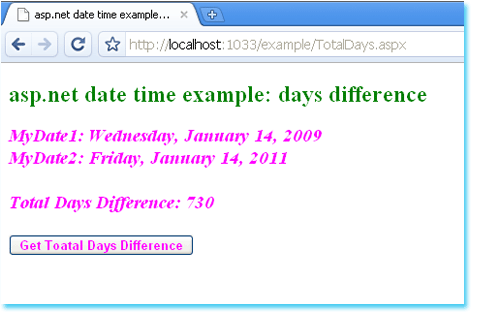### c# - How to get days between two DateTimes

Days between two DateTimes
The following asp.net c# example code demonstrate us how can we get total days difference between two DateTime objects programmatically at run time in an asp.net application.

DateTime.Subtract(DateTime) method allow us to subtract the specified date and time from this instance. We just need to pass a DateTime object as parameter to subtract from specified DateTime object. DateTime.Subtract(DateTime) method return a System.TimeSpan type value. This TimeSpan value represents a time interval that is equal to the date and time of instance minus date and time of parameter.

TimeSpan.TotalDays property get the value of the current TimeSpan structure expressed in whole and fractional days.

TimeSpan.Days property get the days component of the time interval represented by the current TimeSpan structure. This property does not return any fractional day, it just return full day part from a TimeSpan object. Result can be negative or positive number.

So to get the days between two datetimes, the procedure is; first subtract the two DateTime objects. Next, convert the return TimeSpan object to number of days by using TimeSpan.TotalDays property or TimeSpan.Days property. Finally, we will get the days difference between two date time objects.
TotalDays.aspx
``````
<%@ Page Language="C#" %>

<!DOCTYPE html PUBLIC "-//W3C//DTD XHTML 1.0 Transitional//EN" "http://www.w3.org/TR/xhtml1/DTD/xhtml1-transitional.dtd">

<script runat="server">
protected void Button1_Click(object sender, System.EventArgs e) {
DateTime myDate1 = DateTime.Now;
DateTime myDate2 = DateTime.Now.AddYears(2);
TimeSpan difference = myDate2.Subtract(myDate1);

double totalDays = difference.TotalDays;

Label1.Text = "MyDate1: " + myDate1.ToLongDateString();
Label1.Text += "<br />MyDate2: " + myDate2.ToLongDateString();
Label1.Text += "<br /><br />Total Days Difference: " + totalDays;
}
</script>

<html xmlns="http://www.w3.org/1999/xhtml">
<title>asp.net date time example: how to get total days difference between two date time object</title>
<body>
<form id="form1" runat="server">
<div>
<h2 style="color:Green">asp.net date time example: days difference</h2>
<asp:Label
ID="Label1"
runat="server"
Font-Size="Larger"
Font-Italic="true"
Font-Bold="true"
ForeColor="Magenta"
>
</asp:Label>
<br /><br />
<asp:Button
ID="Button1"
runat="server"
Font-Bold="true"
ForeColor="Magenta"
OnClick="Button1_Click"
Text="Get Toatal Days Difference"
/>
</div>
</form>
</body>
</html>
``````More c# examples